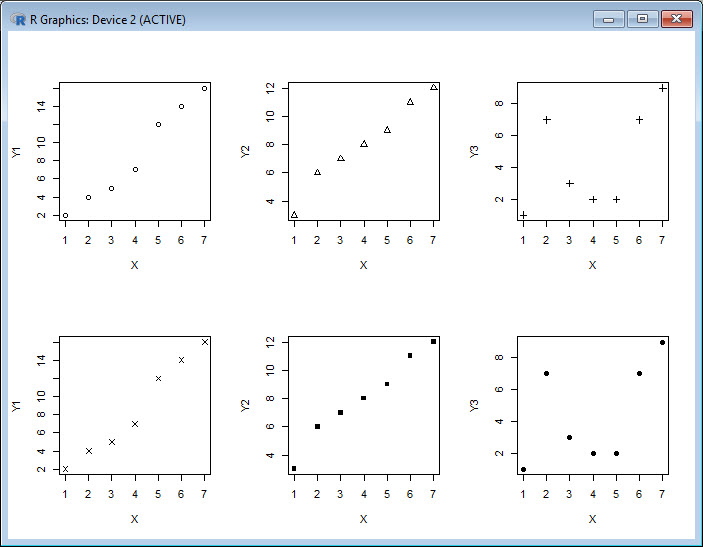# Quick start with R: Plotting multiple graphs on the same page (Part 21)

Today we see how to set up multiple graphs on the same page. We use the syntax `par(mfrow=(A,B))`, where `A` refers to the number of rows and `B` to the number of columns (and where each cell will hold a single graph). This syntax sets up a plotting environment of `A` rows and `B` columns.
First we create four vectors, all of the same length.
`X <- c(1, 2, 3, 4, 5, 6, 7)`
`Y1 <- c(2, 4, 5, 7, 12, 14, 16)`
`Y2 <- c(3, 6, 7, 8, 9, 11, 12)`
`Y3 <- c(1, 7, 3, 2, 2, 7, 9)`
Now we set up a plotting environment of two rows and three columns (in order to hold six graphs), using `par(mfrow())`
`par(mfrow=c(2,3))`
Now we plot six graphs on the same plotting environment. We use the `plot()` command six times in succession, each time graphing one of the `Y` vectors against the `X` vector.
```plot(X,Y1, pch = 1) plot(X,Y2, pch = 2) plot(X,Y3, pch = 3) plot(X,Y1, pch = 4) plot(X,Y2, pch = 15) plot(X,Y3, pch = 16)```
Out plot plot looks like this:That wasn’t so hard! In Blog 22 we will look at further plotting techniques in R.
See you later!
David

#### Annex: R codes used

```# Create four vectors of the same length.
X <- c(1, 2, 3, 4, 5, 6, 7)
Y1 <- c(2, 4, 5, 7, 12, 14, 16)
Y2 <- c(3, 6, 7, 8, 9, 11, 12)
Y3 <- c(1, 7, 3, 2, 2, 7, 9)

# Set up multiple graphs (2 x 3) on the same page.
par(mfrow=c(2,3))

# Create six scatterplots.
plot(X,Y1, pch = 1)
plot(X,Y2, pch = 2)
plot(X,Y3, pch = 3)
plot(X,Y1, pch = 4)
plot(X,Y2, pch = 15)
plot(X,Y3, pch = 16)
```